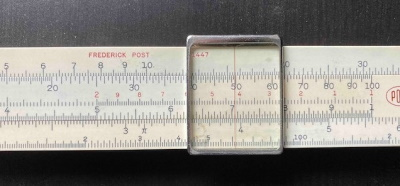# Why a slide rule works

Suppose you have two sticks. The length of one is log x, and the length of the other is log y. If you put the two sticks end to end, the combined length is

log x + log y = log xy.

That’s the basic idea behind a slide rule.

The simplest slide rule consists of two bars with numbers marked on each bar on a logarithmic scale, running from 1 to 10 or maybe 1 to 100.

To multiply x by y, line the 1 on the left end of the bottom bar with x on the top bar. Then the number above y on the bottom bar is xy on the top bar.

This works because the 1 of the bottom bar is positioned a distance log x from the 1 on the top bar. The y on the bottom bar is a distance log y further to the right, and so it is a distance log x + log y from the 1 on the top, which is marked with log xy.

Here’s an example. Suppose you want to find the circumference of a circle 5000 ft in diameter. The image below shows how you would multiply π times 5000.Notice that the 1 of the bottom is under π on the top bar. The red hairline helps us see just where the five on the bottom scale lines up with the top scale, and that’s at about 15.8. Slide rules will only give you the significand (mantissa) of your product, so you have to know that your result is 15,800 ft.

(The lack of exponents could be a pedagogical feature rather than a bug. A few days of using a slide rule would make students think a little harder about what a reasonable answer should be, and would cure them of reporting a ridiculous amount of extraneous precision.)

It’s also possible to line the 10 (or 100) of the right end of the bottom scale with the x value on the top scale.In the image above, I’ve lined the 100 of the bottom scale with 31.4 (10π) on the top scale. We’re only after the significand, so we’ll toss around factors of 10 willy nilly. The number above the 50 on the lower scale is about 15.6 on the upper scale. So this time we’d get an answer of about 15,600 ft. You can see right away that you’re going to need to be more careful, or preferably have a larger slide rule, if you want to get three or four significant figures.

The second example takes a little more effort to understand than the first example. Why can you use the right end of the lower scale?

The 100 position on the lower scale is lined up with 31.4 on the top scale. Where’s the red line? It’s a distance of log 100 – log 50 to the left. So its position on the top scale is

log 31.4 – (log 100 – log 50) = log 31.4 + log 50 – log 100 = log (3.14×5)

and so it’s in the same position as before.

See the next post for an explanation of how a circular slide rule works.